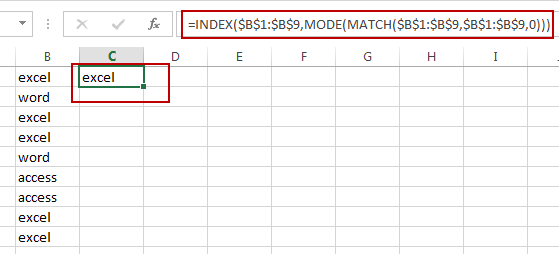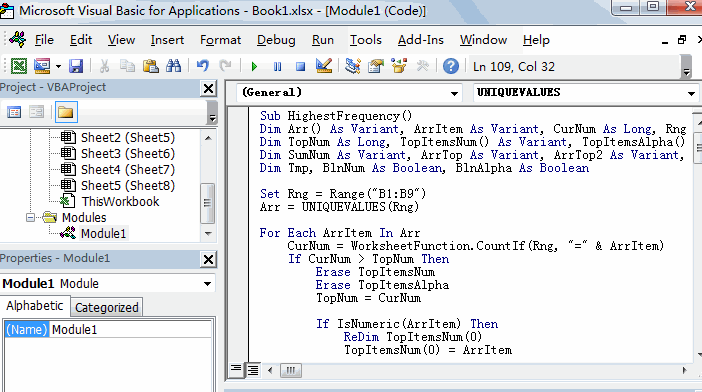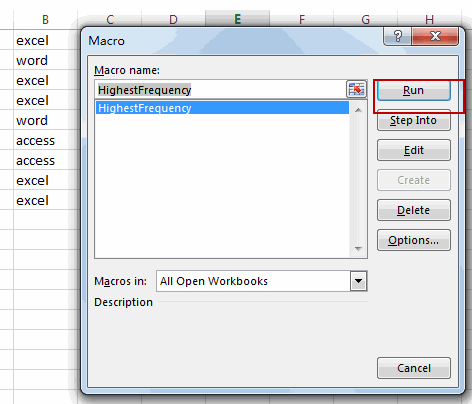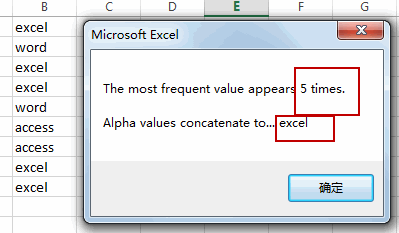## How to Find Most Common Value in a Range in Excel

This post will guide you how to find the most common value in a column of data in Excel. How do I find the most frequently occurring text from a list with array formula in Excel. How to extract the most frequent value from a list of data with VBA Macro in Excel.

## Find Most Common Value with Formula

Assuming that you have a list of data in range B1:B9, in which contain product names. And you need to find the most common text value from the range of cells B1:B9. How to do it. You can use an Excel array formula based on the INDEX function, the MODE function and the MATCH function to extract the most common value from a given range of cells. Like this:

`=INDEX(\$B\$1:\$B\$9,MODE(MATCH(\$B\$1:\$B\$9,\$B\$1:\$B\$9,0)))`

Type this formula into a blank cell and press Shift + Ctrl + Enter keys on your keyboard to apply this array formula.You would notice that the most common value is extracted.

## Find Most Common Value with VBA

You can also use an Excel VBA Macro to achieve the same result of extracting the most common value from a selected range of cells. Here are the steps:

#1 open your excel workbook and then click on “Visual Basic” command under DEVELOPER Tab, or just press “ALT+F11” shortcut.#2 then the “Visual Basic Editor” window will appear.

#3 click “Insert” ->”Module” to create a new module.#4 paste the below VBA code (get code from here) into the code window. Then clicking “Save” button.```Sub HighestFrequency()
Dim Arr() As Variant, ArrItem As Variant, CurNum As Long, Rng As Range
Dim TopNum As Long, TopItemsNum() As Variant, TopItemsAlpha() As Variant
Dim SumNum As Variant, ArrTop As Variant, ArrTop2 As Variant, strConcat As String
Dim Tmp, BlnNum As Boolean, BlnAlpha As Boolean

Set Rng = Range("B1:B9")
Arr = UNIQUEVALUES(Rng)

For Each ArrItem In Arr
CurNum = WorksheetFunction.CountIf(Rng, "=" & ArrItem)
If CurNum > TopNum Then
Erase TopItemsNum
Erase TopItemsAlpha
TopNum = CurNum

If IsNumeric(ArrItem) Then
ReDim TopItemsNum(0)
TopItemsNum(0) = ArrItem
Else
ReDim TopItemsAlpha(0)
TopItemsAlpha(0) = ArrItem
End If

ElseIf CurNum = TopNum Then

If IsNumeric(ArrItem) Then
On Error Resume Next
ReDim Preserve TopItemsNum(UBound(TopItemsNum) + 1)
If Err.Number <> 0 Then ReDim TopItemsNum(0)
Err.Clear
On Error GoTo 0
TopItemsNum(UBound(TopItemsNum)) = ArrItem
Else
On Error Resume Next
ReDim Preserve TopItemsAlpha(UBound(TopItemsAlpha) + 1)
If Err.Number <> 0 Then ReDim TopItemsAlpha(0)
Err.Clear
On Error GoTo 0
TopItemsAlpha(UBound(TopItemsAlpha)) = ArrItem
End If
End If
Next ArrItem

On Error Resume Next
Tmp = TopItemsNum(0)
If Err.Number <> 0 Or Tmp = "" Then GoTo Skip
On Error GoTo 0

For Each ArrTop In TopItemsNum
BlnNum = True
SumNum = ArrTop + SumNum
Next ArrTop

Skip:
On Error Resume Next
Tmp = TopItemsAlpha(0)
If Err.Number <> 0 Or Tmp = "" Then GoTo Skip2
On Error GoTo 0

For Each ArrTop2 In TopItemsAlpha
If Err.Number <> 0 Then Exit For
BlnAlpha = True
strConcat = ArrTop2 & strConcat
Next ArrTop2
On Error GoTo 0

Skip2:

If BlnNum = True And BlnAlpha = True Then
MsgBox "The most frequent value appears " & TopNum & " times." & vbLf & vbLf & _
"Numeric values average to... " & SumNum & vbLf & vbLf & _
"Alpha values concatenate to... " & strConcat
ElseIf BlnNum = True Then
MsgBox "The most frequent value appears " & TopNum & " times." & vbLf & vbLf & _
"Numeric values average to... " & SumNum
Else
MsgBox "The most frequent value appears " & TopNum & " times." & vbLf & vbLf & _
"Alpha values concatenate to... " & strConcat
End If

End Sub

Function UNIQUEVALUES(ParamArray Target() As Variant) As Variant
Dim UniqueCollection As New Collection, TmpArr() As Variant
Dim Rng As Range, vItem As Variant, Cell As Range

Application.Volatile

'Merge the target ranges into one range
For Each vItem In Target
If Rng Is Nothing Then
Set Rng = vItem
Else
Set Rng = Application.Union(Rng, vItem)
End If
Next vItem

'loop through range and create 1D array
For Each Cell In Rng
On Error Resume Next

If Err.Number = 0 Then 'if already in collection an error will occur
ReDim Preserve TmpArr(UBound(TmpArr) + 1)
If Err.Number <> 0 Then ReDim TmpArr(0)
On Error GoTo 0

TmpArr(UBound(TmpArr)) = Cell.Value
End If

Err.Clear
Next Cell

'Return result. If a single column transpose array.
UNIQUEVALUES = TmpArr

End Function```

#5 back to the current worksheet, then run the above excel macro. Click Run button.#6 Let’ see the result:### Related Functions

• Excel INDEX function
The Excel INDEX function returns a value from a table based on the index (row number and column number)The INDEX function is a build-in function in Microsoft Excel and it is categorized as a Lookup and Reference Function.The syntax of the INDEX function is as below:= INDEX (array, row_num,[column_num])…
• Excel MATCH  function
The Excel MATCH function search a value in an array and returns the position of that item.The MATCH function is a build-in function in Microsoft Excel and it is categorized as a Lookup and Reference Function.The syntax of the MATCH function is as below:= MATCH  (lookup_value, lookup_array, [match_type])….